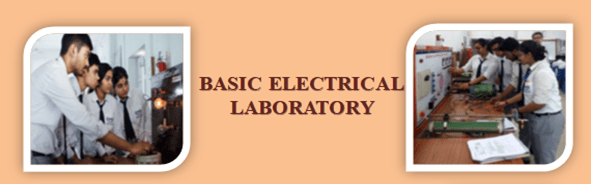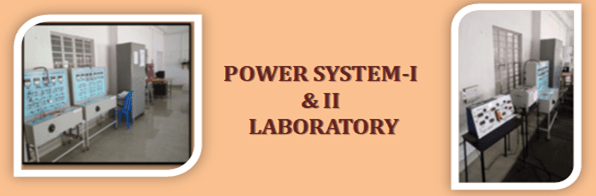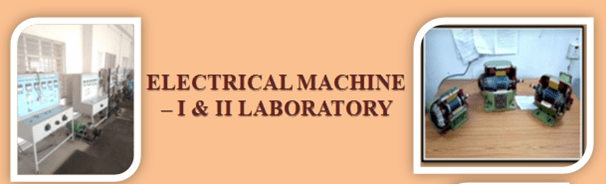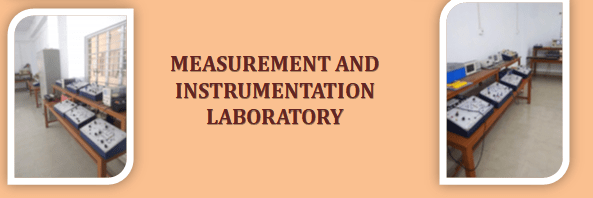# Laboratories### LIST OF EXPERIMENTS IN LABORATORIES

Basic Electrical Engineering Laboratory

1. To study the characteristics of carbon & tungsten filament lamp.
2. To study the characteristics of fluorescent lamp.
3. Verification of superposition theorem.
4. Verification of thevenin’s theorem.
5. Verification of maximum power transfer theorem.
6. Study of RLC series circuit.
7. Study of RLC parallel circuit.
8. Speed control of dc shunt motor.
9. Measurement of 3-phase power by two wattmeter method.
10. Equivalent circuit of single phase transformer.

Electrical Circuit Analysis Laboratory

1. Transient response of R-L network: simulation with PSPICE /Hardware
2. Transient response of R-C network: simulation with PSPICE /Hardware
3. Transient response of R-L-C series circuit: Simulation with PSPICE/ Hardware
4. Transient response of R-L-C parallel circuit: Simulation with PSPICE/ Hardware
5. Determination of Impedance (Z) and Admittance (Y) parameter of two port network in T network: Simulation / Hardware.
6. Determination of Impedance (Z) and Admittance (Y) parameter of two port network in π network: Simulation / Hardware.
7. Frequency response of LP and HP filters: Simulation / Hardware.
8. Frequency response of BP and BR filters: Simulation /Hardware.
9. Generation of Periodic, Exponential, Sinusoidal, Damped Sinusoidal, Step, Impulse, Ramp signal using MATLAB in both discrete and analog form.
10. Determination of Laplace transform and Inverse Laplace transform using MATLAB.
11. Verification of Thevenin, and Norton Theorem using PSpice / Hardware
12. Verification of Superposition Theorem using PSpice / Hardware

Analog Electronics Laboratory

1. Study of Ripple and Regulation characteristics of full wave rectifier without capacitor filter.
2. Study of Ripple and Regulation characteristics of full wave rectifier with capacitor filter.
3. Study of Zener diode as voltage regulator
4. Construction of two stage R-C coupled amplifier & study of its gain and Bandwidth.
5. Study of class A amplifier
6. Study of class C amplifier.
7. Realization V-I converter using Operational Amplifier.
8. Realization I-V converter using Operational Amplifier
9. Study of timer circuit using NE 555 and configuration of Monostable Multivibrator.
10. Study of timer circuit using NE 555 and configuration of Astable Multivibrator
11. Study of DAC

ELECTRIC MACHINE LABORATORY-I

1. Study of the characteristics of a separately excited DC generator.
2. Study of the characteristics of a DC motor.
3. Study of methods of speed control of DC motor.
4. Study of the characteristics of a compound DC generator (short shunt).
5. Measurement of speed of DC series motor as a function of load torque.
6. Open circuit test of a single phase transformer.
7. Short circuit test of a single phase transformer
8. Demonstration of different types of motor
9. Polarity test on a single phase transformer & study of different connections of three phase transformer.
10. Study of equivalent circuit of three phase Induction motor by no load and blocked rotor test.
11. Study of performance of wound rotor Induction motor under load.
12. Study of performance of three phase squirrel- cage Induction motor –determination of iron- loss, friction & windage loss

DIGITAL ELECTRONICS LABORATORY

1. Study of Basic Gates
2. Realisation of basic gates using Universal logic gates.
3. Realisation of RS flip flop using logic gates.
4. Realisation of JK flip flop using logic gates
5. Realisation of D flip flop using logic gates
6. Design of Combinational circuit for BCD to decimal conversion to drive 7-segment display using Multiplexer.
7. Realisation of Synchronous Up counters.
8. Realisation of Synchronous Down counter
9. Construction of simple Decoder & Multiplexer circuits using logic gates.
10. Construction of simple Multiplexer circuits using logic gates
11. Construction of adder circuit using Shift register

MEASUREMENT AND INSTRUMENTATION LABORATORY

1. Instrument workshop- Observe the construction of PMMC, Dynamometer, Electrothermal and Rectifier type of instruments, Oscilloscope and Digital multimeter.
2. Calibrate moving iron type ammeter/voltmeter by potentiometer.
3. Calibrate electrodynamometer type ammeter/voltmeter by potentiometer.
4. Calibrate dynamometer type wattmeter by potentiometer.
5. Calibrate AC energy meter.
6. Measurement of resistance using Kelvin double bridge.
7. Measurement of power using Instrument transformer.
8. Measurement of power in Polyphase circuits.
9. Measurement of frequency by Wien Bridge.
10. Measurement of Inductance by Anderson bridge
11. Measurement of capacitance by De Sauty Bridge.
12. Measurement of capacitance by Schering Bridge.

ELECTRICAL MACHINES-II LABORATORY

1. Different methods of starting of a 3 phase Cage Induction Motor & their comparison [DOL, Auto transformer & Star-Delta]
2. Speed control of 3 phase squirrel cage induction motor by different methods & their comparison [voltage control & frequency control].
3. Speed control of 3 phase slip ring Induction motor by rotor resistance control.
4. Determination of regulation of Synchronous machine by
• Potier reactance method.
• Synchronous Impedance method.
5. Determination of equivalent circuit parameters of a single phase Induction motor.
6. Load test on single phase Induction motor to obtain the performance characteristics.
7. To determine the direct axis resistance [Xd] & quadrature reactance [Xq] of a 3 phase synchronous machine by slip test.
8. Load test on wound rotor Induction motor to obtain the performance characteristics.
9. To make connection diagram to full pitch & fractional slot winding of 18 slot squirrel cage Induction motor for 6 poles & 4 pole operation.
10. To study the performance of Induction generator.
11. Parallel operation of 3 phase Synchronous generators.
12. V-curve of Synchronous motor

POWER SYSTEMS LABORATORY – I

1. Overview of the different components of power system
2. Determination of the generalized constants A.B, C, D of long transmission line.
3. Simulation of DC distribution by network analyzer.
4. Measurement of earth resistance by earth tester.
5. Dielectric strength test of insulating oil.
6. Determination of breakdown strength of solid insulating material.
7. Different parameter calculation by power circle diagram
8. Study of different types of insulator.
9. Active and reactive power control of alternator.
10. Study and analysis of an electrical transmission line circuit with the help of PSPICE.
11. Dielectric constant, tan delta, resistivity test of transformer oil.
12. Overview of Different Faults in the power system

CONTROL SYSTEMS LABORATORY

1. Familiarization with MAT-Lab control system tool box, MAT-Lab- simulink tool box & PSPICE
2. Determination of Step response for first order system with unity feedback on CRO & calculation of control system specification like Time constant, % peak overshoot, settling time etc. from the response.
3. Determination of Step response for Second order system with unity feedback on CRO & calculation of control system specification like Time constant, % peak overshoot, settling time etc. from the response
4. Simulation of Step response & Impulse response for type-0, type-1 & Type-2 system with unity feedback using MATLAB & PSPICE.
5. Determination of Root locus using MATLAB control system tool box for 2nd order system & determination of different control system specification from the plot.
6. Determination of Bode plot using MATLAB control system tool box for 2nd order system & determination of different control system specification from the plot.
7. Determination of Nyquist plot using MATLAB control system tool box for 2nd order system & determination of different control system specification from the plot.
8. Determination of PI controller action of first order simulated process.
9. Determination of PD controller action of first order simulated process
10. Determination of PID controller action of first order simulated process
11. Determination of approximate transfer functions experimentally from Bode plot.
12. Evaluation of steady state error, setting time, percentage peak overshoot, gain margin, phase margin with addition of Lead

MICROPROCESSORS LABORATORY

1. Familiarization with 8085 register level architecture and trainer kit components including the memory map. Familiarization with the process of storing and viewing the contents of memory as well as registers.
2. (a) Study of prewritten program on trainer kit using the basic instruction set ( data transfer, load/store, arithmetic, logical)

(b) Assignment based on that.
3. (a) Familiarization with 8085 simulator on PC

(b) Study of prewritten program using basic instruction set (data transfer, load/store, arithmetic, logical).

(c) Assignment based on that.
4. Programming using kit/simulator.
• Lookup table
• Copying a block of memory
• Shifting a block of memory.
• Packing and unpacking of BCD numbers.
• Binary to ASCII conversion
• String matching
5. Program using subroutine calls and using IN/OUT instruction using 8255 PPI on the trainer kit e.g. subroutine for delay, reading switch state and glowing LEDs accordingly, finding out frequency of pulse train etc.
6. Interfacing any 8 bit latch (74LS373) with trainer kit as a peripheral mapped output port with absolute address decoding.
7. Interfacing with I/O module :
• Speed control of DC motor with DAC
• Keyboard
• Multi digit display with multiplexing.
• Stepper motor

POWER SYSTEM-II LABORATORY

1. Study of the characteristics of on delay relay and off delay relay.
2. Test to find out polarity, ratio and magnetization characteristics of CT and PT.
3. Test to find out characteristics of
• under voltage relay
• earth fault relay.
4. Study on DC load flow
5. Study on AC load flow using Gauss-Seidel method
6. Study on AC load flow using Newton Raphson method.
7. Study on Economic load dispatch.
8. Study of different transformer protection schemes by simulation.
9. Study of different generator protection schemes by simulation.
10. Study of different motor protection schemes by simulation.
11. Study of different characteristics of over current relay.
12. Study of different protection scheme for feeder.

Power Electronics Laboratory

1. Study of the characteristics of an SCR.
2. Study of the characteristics of a Triac
3. Study of different triggering circuits of an SCR
4. Study of firing circuits suitable for triggering SCR in a single phase full controlled bridge.
5. Study of the operation of a single phase full controlled bridge converter with R and R-L load.
6. Study of performance of single phase half controlled symmetrical and asymmetrical bridge converters.
7. Study of performance of step down chopper with R and R-L load.
8. Study of performance of single phase controlled converter with and without source inductance (simulation)
9. Study of performance of step up and step down chopper with MOSFET, IGBT and GTO as switch (simulation).
10. Study of performance of single phase half controlled symmetrical and asymmetrical bridge converter (simulation)
11. Study of performance of three phase controlled converter with R & R-L load. (simulation)
12. Study of performance of PWM bridge inverter using MOSFET as switch with R and R-L load.
13. Study of performance of three phase AC controller with R and R-L load (simulation)
14. Study of performance of a Dual converter. (simulation)
15. Study of performance of a Cycloconverters (simulation)

ELECTRICAL DESIGN LABORATORY

1. Familiarization of synchronous machine with the help of cut section models.
2. Familiarization of single phase induction machine with the help of cut section models
3. Familiarization of three phase induction machine with the help of cut section models
4. Familiarization of DC machine with the help of cut section models
5. Familiarization of single phase transformers with the help of cut section models
6. Familiarization of three phase transformers with the help of cut section models
7. Familiarization with the construction of single phase fan.
8. Design & Fabrication of air and iron core inductor.
9. Design & fabrication of small single phase transformer, 100 VA, 220/12 V.
10. Design & fabrication of 10 W wire wound resistor.
11. Introduction to computer aided machine design.